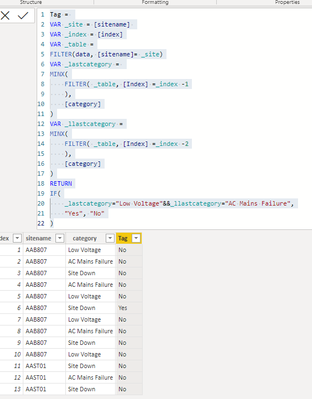cancel
Showing results for
Did you mean:Frequent Visitor

## Compare Previous Two Rows with a Current Row using DAX

Hi Everyone,

I have to create a new column and am trying to compare rows in a single column when a particular value exists in any of the rows. For e.g.

If Table[category] = "Site Down", then check for Table[category row - 1] = "low voltage" && Table[category row - 2] = "AC Mains Failure", "YES" otherwise "NO"

I am unable to access the previous rows in DAX. I've tried using the EARLIER function but am unable to get the exact thing. This is easy to achieve in SQL using LAG() or NEIGHBOR() function.

Sample data link is shared below. Please note that this data is first sorted using 'sitename; and then by 'first occurence' column since it is always expected that low voltage & ac mains failure will come before site down respectively.

Thanks!

1 ACCEPTED SOLUTIONSuper User

There are newer DAX functions that are similar to SQL's LAG. For example, OFFSET:

``````IsSiteDown =
VAR Summary =
SUMMARIZE (
Table2,
Table2[sitename],
Table2[category],
Table2[firstoccurrence]
)
VAR Prev1Cat =
MAXX (
OFFSET (
-1,
Summary,
ORDERBY ( Table2[firstoccurrence] ),
PARTITIONBY ( Table2[sitename] )
),
Table2[category]
)
VAR Prev2Cat =
MAXX (
OFFSET (
-2,
Summary,
ORDERBY ( Table2[firstoccurrence] ),
PARTITIONBY ( Table2[sitename] )
),
Table2[category]
)
VAR Result =
IF (
Table2[category] = "Site Down"
&& Prev1Cat = "Low Voltage"
&& Prev2Cat = "AC Mains Failure",
"Yes",
"No"
)
RETURN
Result``````

8 REPLIES 8Super User

There are newer DAX functions that are similar to SQL's LAG. For example, OFFSET:

``````IsSiteDown =
VAR Summary =
SUMMARIZE (
Table2,
Table2[sitename],
Table2[category],
Table2[firstoccurrence]
)
VAR Prev1Cat =
MAXX (
OFFSET (
-1,
Summary,
ORDERBY ( Table2[firstoccurrence] ),
PARTITIONBY ( Table2[sitename] )
),
Table2[category]
)
VAR Prev2Cat =
MAXX (
OFFSET (
-2,
Summary,
ORDERBY ( Table2[firstoccurrence] ),
PARTITIONBY ( Table2[sitename] )
),
Table2[category]
)
VAR Result =
IF (
Table2[category] = "Site Down"
&& Prev1Cat = "Low Voltage"
&& Prev2Cat = "AC Mains Failure",
"Yes",
"No"
)
RETURN
Result``````Frequent Visitor

@AlexisOlson Yes It works. Thanks a lot!

Could you please explain the use of MAXX? Could it be any other iterator?Super User

OFFSET returns a row and I want a specific column from that row, so I selected it using MAXX. There isn't anything special about MAXX; you could use other iterators too. SELECTCOLUMNS would work too and is probably more intuitive.Community Champion

you would need to
1) add an index column in Power Query

2) add a new column with DAX like this:

``````Tag =
VAR _site = [sitename]
VAR _index = [index]
VAR _table =
FILTER(data, [sitename]= _site)
VAR _lastcategory =
MINX(
FILTER( _table, [Index] =_index -1
),
[category]
)
VAR _llastcategory =
MINX(
FILTER( _table, [Index] =_index -2
),
[category]
)
RETURN
IF(
[category]="Site Down"
&&_lastcategory="Low Voltage"
&&_llastcategory="AC Mains Failure",
"Yes", "No"
)``````

i tried and it worked like this:Frequent Visitor

@FreemanZ thanks for your reply. Your solution seems to work when I add the index using the sitename & category column, however with all the other columns mentioned in the sample data file it is giving 'No' for all the rows. Could we specify a column when trying to add an index column?Community Champion

1) Power BI is efficient handling columns but not that good handling rows.

2) EARLIER is to get the row context in the earlier set of iteration, not the context of the earlier row or the earlier iteration scanning in the current set of iteration.Super User

If you are going by firstoccurence to establish an order and look for the previous rows, what happens when the datetime in firstoccurence is exactly the same? This happens several times in your sample data. One option would be to sort as required in PQ and add an index column, then base it all on that index instead of on firstoccurence Pending that, try this for your column. See it all at work in the attached file.

``````NewColumn =
VAR previous_ =
CALCULATE (
MAX ( Table1[firstoccurrence] ),
Table1[firstoccurrence] < EARLIER ( Table1[firstoccurrence] ),
ALLEXCEPT ( Table1, Table1[sitename] )
)
VAR previous2Prev_ =
CALCULATE (
MAX ( Table1[firstoccurrence] ),
Table1[firstoccurrence] < previous_,
ALLEXCEPT ( Table1, Table1[sitename] )
)
VAR catPrevious_ =
CALCULATE (
MAX ( Table1[category] ),
Table1[firstoccurrence] = previous_,
ALLEXCEPT ( Table1, Table1[sitename] )
)
VAR catPrevious2Prev_ =
CALCULATE (
MAX ( Table1[category] ),
Table1[firstoccurrence] = previous2Prev_,
ALLEXCEPT ( Table1, Table1[sitename] )
)
RETURN
IF (
Table1[category] = "Site Down",
IF (
catPrevious_ = "Low Voltage"
&& catPrevious2Prev_ = "AC Mains Failure",
"Yes",
"No"
)
)``````Please accept the solution when done and consider giving a thumbs up if posts are helpful.  Contact me privately for support with any larger-scale BI needs, tutoring, etc.Frequent Visitor

@AlB thanks for your reply. it seems to work for small datasets but for the actual data (>50k+ rows) it crashed. However alxexis's answer seems to be the most comprehensive one!